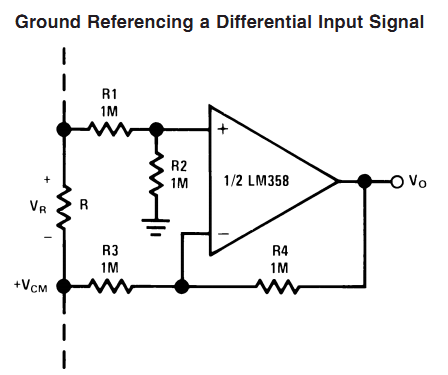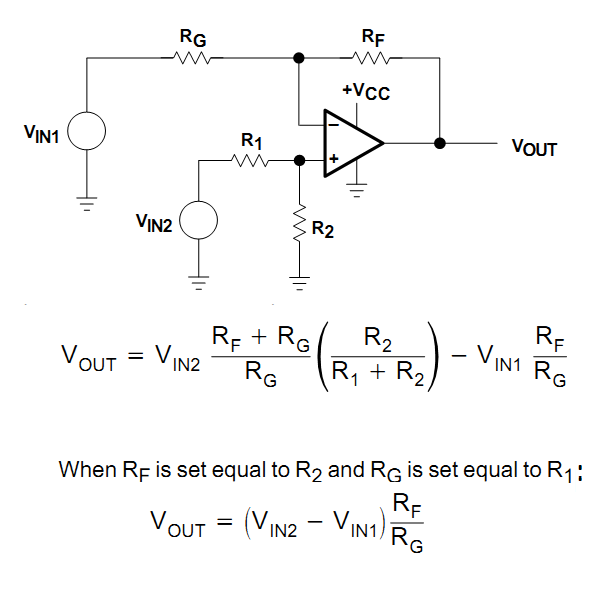# Problem About LM358 to make Difference Amplifier

Status
Not open for further replies.

##### Junior Member level 3
Hi, I want to use LM-358 as Difference Amplifier.
I want to take Input V1 & V2 then my output will be Vo=V1-V2.
Please give me the circuit Diagram of Difference Amplifier with IC-LM358.

You can find the circuit in the datasheet (attached).

This is the circuit from the datasheet (unity gain):Hope this helps.
Tahmid.

Attachment replaced with link, don’t upload files that are widely available in the internet and can be found easily like datasheets or application notes, this is a waste of valuable bandwidth, post a link instead.

LM358 datasheet

Last edited by a moderator:
•Points: 2

Brother, is there any options to convert Current --> Voltage.
If yes, what is the available in our local market.

Just use a sense resistor.

V = I * R

Voltage across resistor is equal to the product of current through resistor and resistance of resistor.

Hope this helps.
Tahmid.

•Points: 2
Sense resistor?
In market what should i say them if i want to buy it?
Also, How can i operate it. How can I take output from sense resistor?

Also, I want to inform you that i went to patuatuli for buying current sensor. Sellers gave me many(5 to 6 models of current sensor) information about Current Sensor. But I could not take any decision which sensor should I use? cause I don't got any reliable information about the models. Brother If I want to use current sensor what model should i use. Please if you know inform me about the current sensor by giving model number/numbers.
Thanks

You can get quite a few hall-effect sensors. These are quite easy to use and efficient. However, they are expensive and are not required if high accuracy/precision is not required.

You can use a regular resistor. It's called sense resistor because it is used for current sensing.
First you need to figure out resistance required. Then, you need to figure out required power.

What is the current level you are trying to measure and what is the voltage across it that you require?

You can use 0.1Ω, 0.01Ω, 0.001Ω, etc for current sensing, depending on required current sensing and power dissipation. I know that you can get down to 0.01Ω in the local market.

For selecting resistance and power rating, make use of the two equations:

V = I * R

P = ${ I}^{2 }$ * R

Hope this helps.
Tahmid

•Points: 2
Thanks Brother,
1)
I want to charge 12V, 7.2Ah Lead Acid battery'
So my current near equal to 0-10Ah.
Now, What resistor I Should use?
2)
Please give me the circuit diagram to sense current using "sense resistor" ,also i want to drive such circuit with only +Ve bias voltage.

If you're charging a 7.2Ah battery, you shouldn't be charging it at more than 1A.

If you use a 1Ω resistor, voltage across the resistor will be 1V at 1A. Power dissipation will be 1W at 1A.

If you use a 0.1Ω resistor, voltage across the resistor will be 0.1V at 1A. Power dissipation will be 0.1W at 1A.

Use the resistor between the battery -ve terminal and the charging supply -ve. Make use of the voltage across the resistor. If the opamp/comparator ground is the charging supply ground, a simple comparator (with non-inverting amplifier if required) will suffice.

You can place the resistor in the +ve line instead and use a difference amplifier and then a comparator.

Hope this helps.
Tahmid.

Status
Not open for further replies.